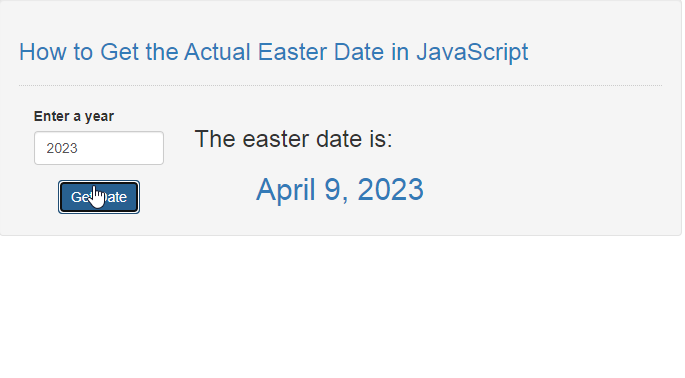# How to Get the Actual Easter Date in JavaScript

## Introduction

In this tutorial we will create a How to Get the Actual Easter Date in JavaScript. This tutorial purpose is to teach you how to get the actual date for the easter day. This will tackle all the basic function that will display the easter date. I will provide a sample program to show the actual coding of this tutorial.

This tutorial is simple and easy to understand just follow the instruction I provided and you can do it without a problem. This program can be use to any system or application if you want to display some important like the easter day. I will give my best to provide you the easiest way of creating this program Display the Easter Date. So let's do the coding.

## Before we get started:

This is the link for the template that i used for the layout design https://getbootstrap.com/.

## Creating The Interface

This is where we will create a simple interface for our application. This code will display html forms and button for display easter date. To create this simply copy and write it into your text editor, then save it as index.html.
`<!DOCTYPE html><html lang="en">	<head>		<meta charset="UTF-8" name="viewport" content="width=device-width, initial-scale=1"/>		<link rel="stylesheet" type="text/css" href="css/bootstrap.css" />	</head><body>	<nav class="navbar navbar-defalt">		<div class="container-fluid">			<a class="navbar-brand" href="https://sourcecodester.com">Sourcecodester</a>		</div>	</nav>	<div class="col-md-3"></div>	<div class="col-md-6 well">		<h3 class="text-primary">How to Get the Actual Easter Date in JavaScript</h3>		<hr style="border-top:1px dotted #ccc;"/>		<div class="col-md-3">			<form>				<div class="form-group">					<label>Enter a year</label>					<input type="number" id="year" class="form-control"/>				</div>				<center><button type="button" onclick="displayDate();" class="btn btn-primary">Get Date</button></center>			</form>		</div>		<div class="col-md-6">			<div id="result"></div>		</div>	</div><script src="script.js"></script>	</body>	</html>`

## Creating JavaScript Function

This is where the main function of the application is. This code will try you get the actual easter date base on the entered year. To do this just copy and write these block of codes inside the text editor and save it as script.js.
`let m=["January", "February", "March", "April", "May", "June", "July", "August", "September", "October", "November", "December"];  function displayDate(){	let year=document.getElementById('year').value; 	if(year.length == 0){		alert("Please enter something");	}else{		let month=getEasterDate(year);		let day=getEasterDate(year);		document.getElementById('result').innerHTML="<h3>The easter date is: </h3><center><h2 class='text-primary'>"+m[month-1]+" "+day+", "+year+"</h2></center>";	}} function getEasterDate(year) {	let f = Math.floor;	let G = year % 19;	let	C = f(year / 100);	let H = (C - f(C / 4) - f((8 * C + 13)/25) + 19 * G + 15) % 30;	let I = H - f(H/28) * (1 - f(29/(H + 1)) * f((21-G)/11));	let J = (year + f(year / 4) + I + 2 - C + f(C / 4)) % 7;	let L = I - J;	let month = 3 + f((L + 40)/44);	let day = L + 28 - 31 * f(month / 4); 	return [month,day];}`

In the code above we a method that will calculate the date for the easter day, we will call this getEasterDate(). This function contains several algorithm that will calculate and target the actual easter date. Next method will be called displayDate(), this function purpose is to display the easter date after the calculation process is done.

## Output: